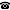# Forcing Axioms and the foundations of mathematics

Talk held by Giorgio Venturi (Scuola Normale Superiore di Pisa, Italy) at the KGRC Friday seminar on 2013‑11‑22.

### Abstract

In this talk I would like to discuss the role of Forcing Axioms in the context of the foundational aspects of set theory. I propose to look at set theory not only as a foundation of mathematics in a traditional sense, but as a foundation for mathematical practice. For this purpose I distinguish between a standard, ontological, set theoretical foundation that aims to find a set-theoretical surrogate to every mathematical object, and a practical one that tries to explain mathematical phenomena, giving necessary and sufficient conditions for the proof of mathematical propositions. I will present some example of this use of set-theoretical methods, in the context of mainstream mathematics, in terms of independence proofs, equiconsistency results and discussing some results, by Woodin and by Viale, that show how it is possible to "complete" respectively the structures $H(\aleph_1)$ and $H(\aleph_2)$. In particular the use of an extension of MM, one of the strongest known Forcing Axioms, in Viale's result, will be shown to be an argument in favor of the accordance of the Forcing Axiom with the foundational aim of set theory; and so in favor of their justification. In the end I will propose a more general distinction between two different kinds of foundation: a practical one and a theoretical one, drawing some examples from the history of the foundation of mathematics.

### References

 Giorgio Venturi: "Foundation of mathematics between theory and practice". Preprint.

 Matteo Viale: "Category forcings, MM$^{+++}$, and generic absoluteness for the theory of strong forcing axioms". Preprint.

Kurt Gödel Research Center for Mathematical Logic. Währinger Straße 25, 1090 Wien, Austria.+43-1-4277-50501. Last updated: 2013-11-26, 07:36.